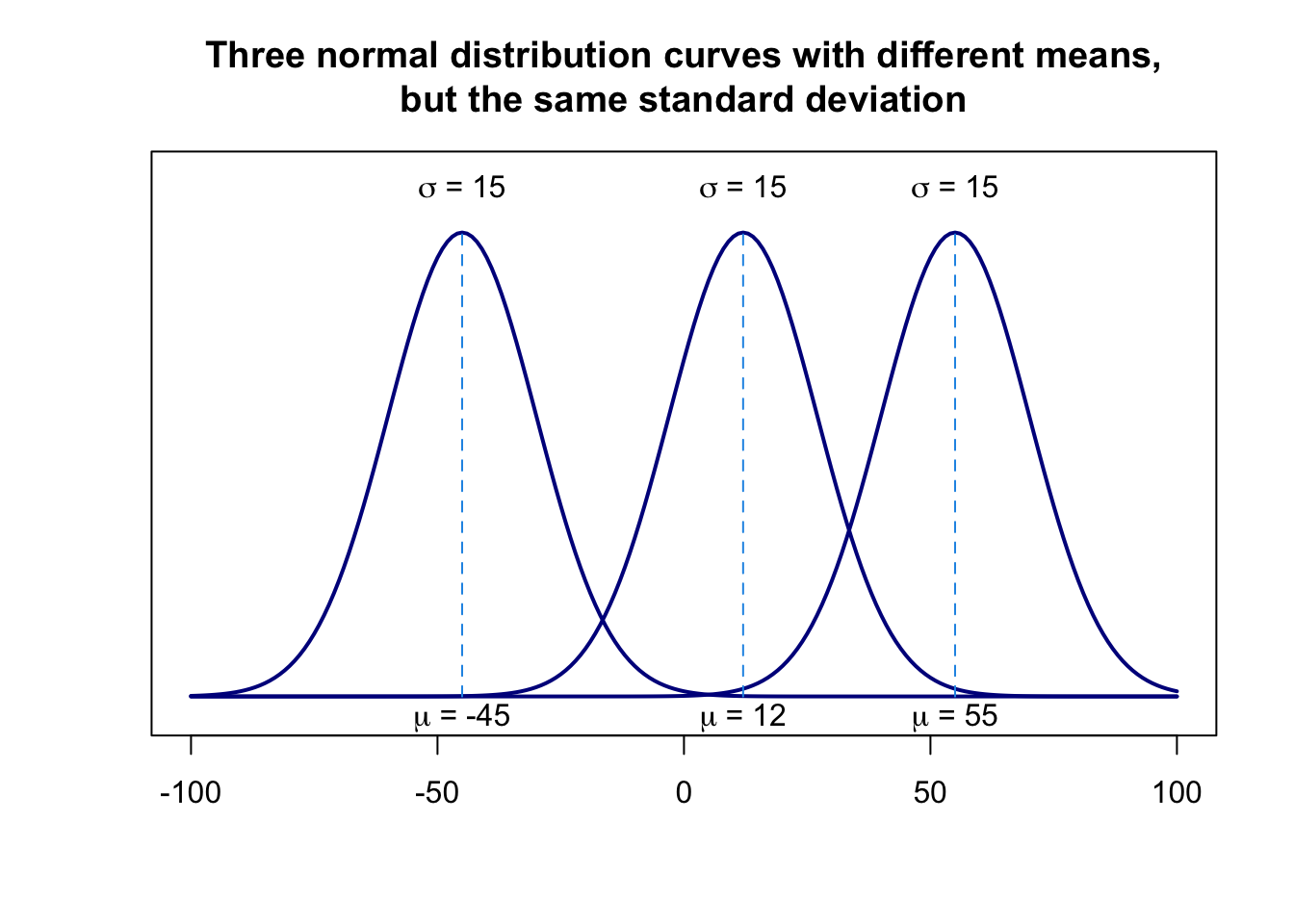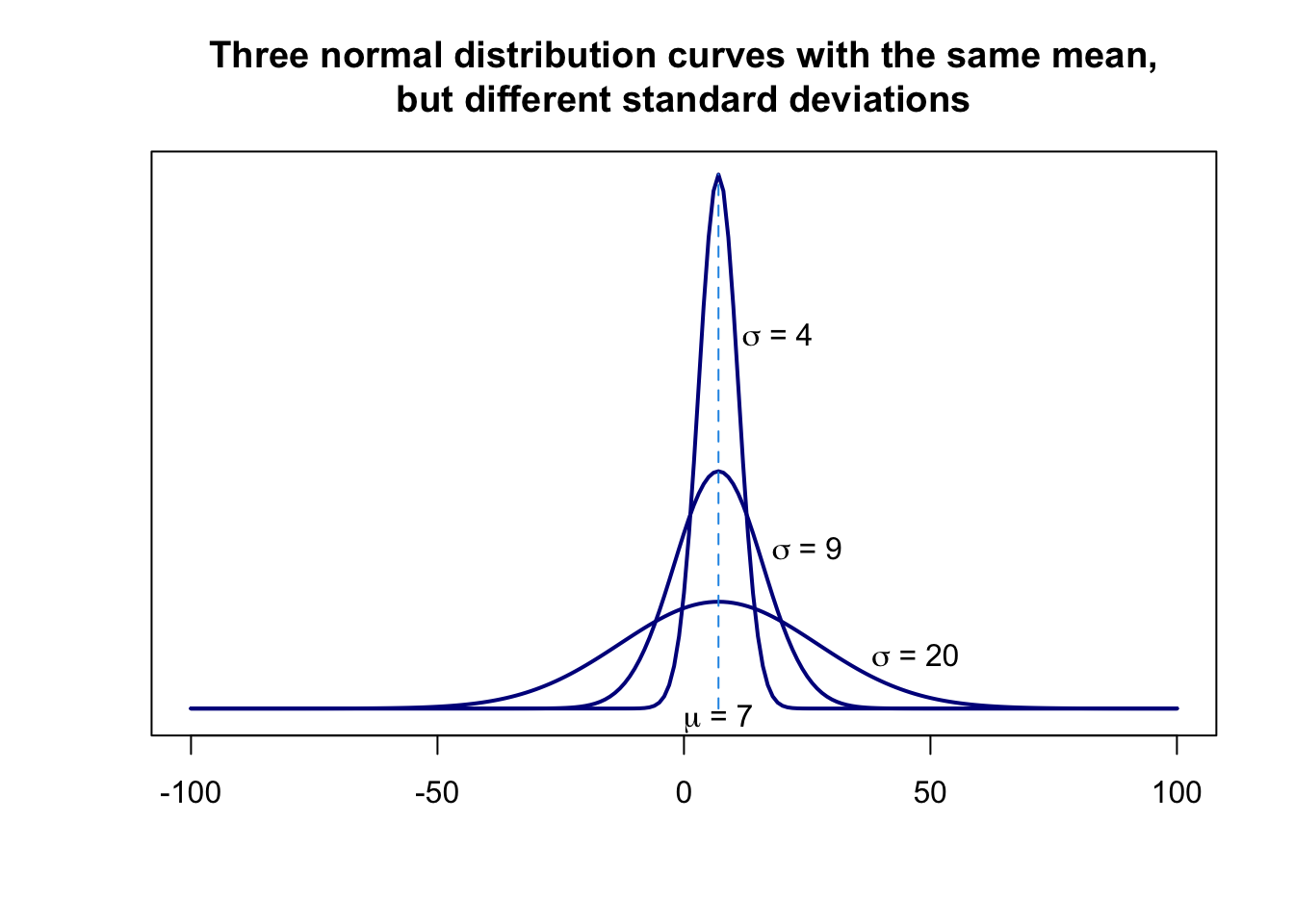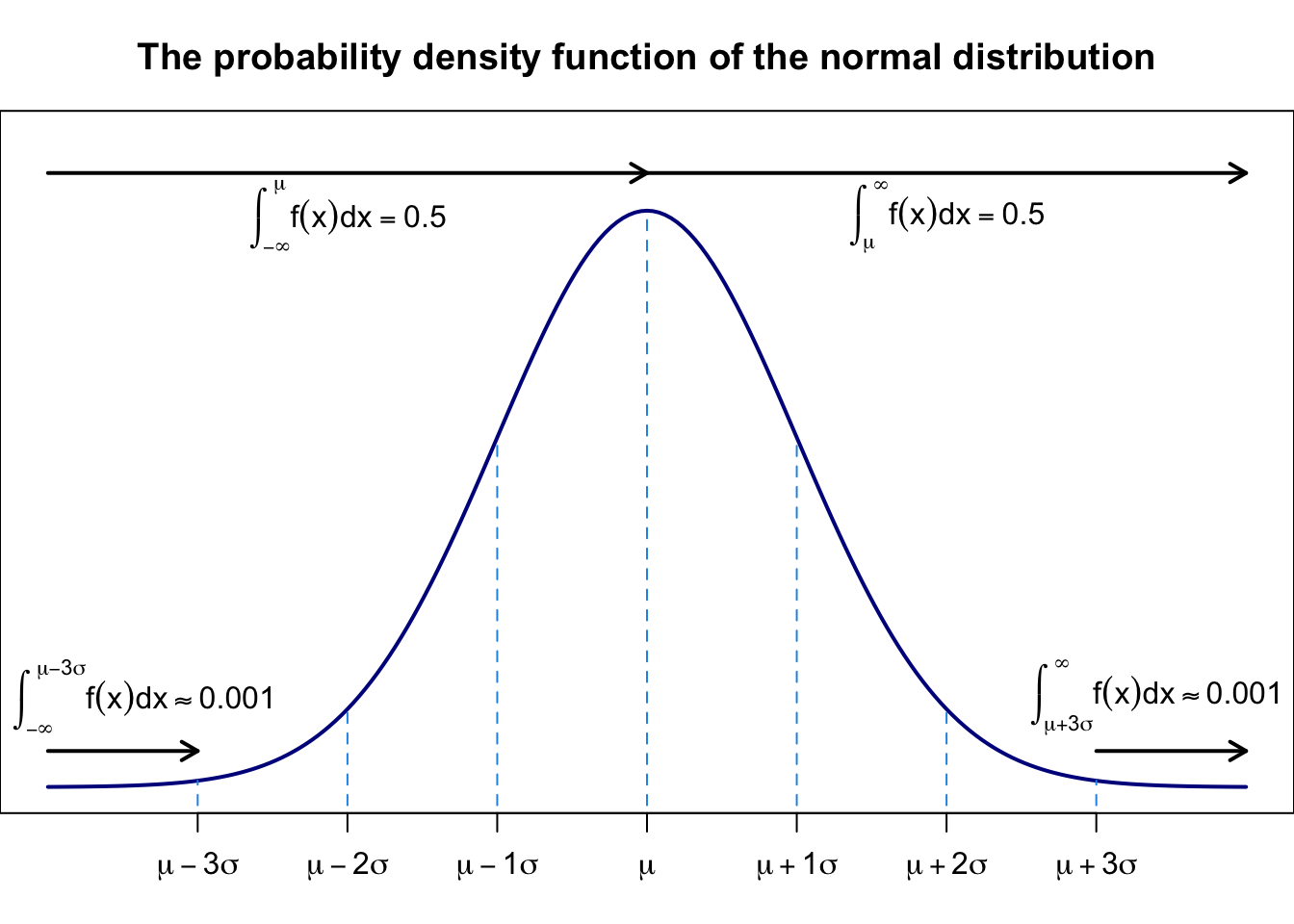The normal distribution is used extensively in probability theory, statistics as well as the natural and social sciences. It is also called the Gaussian distribution because Carl Friedrich Gauss (1777-1855) was one of the first to apply it for the analysis of astronomical data (Lovric 2011).

The normal probability distribution or the normal curve is a bell-shaped (symmetric) curve. Its mean is denoted by $$\mu$$ and its standard deviation by $$\sigma$$. A continuous random variable $$x$$ that has a normal distribution is called a normal random variable.

The notation for a normal distribution is $$X \sim N(\mu,\sigma)$$. The probability density function (PDF) is written as

$f(x) = \frac{1}{\sigma \sqrt{2 \pi}}e^{-\frac{1}{2}\left(\frac{x-\mu}{\sigma}\right)^2}$

where $$e \approx 2.71828$$ and $$\pi \approx 3.14159$$. The probability density function $$f(x)$$ gives the vertical distance between the horizontal axis and the normal curve at point $$x$$.

The normal distribution is described by two parameters, the mean, $$\mu$$, and the standard deviation, $$\sigma$$. Each different set of values of $$\mu$$ and $$\sigma$$ results in a different normal distribution. The value of $$\mu$$ determines the center of a normal distribution curve on the horizontal axis, while the value of $$\sigma$$ determines the spread of the normal distribution curve.A normal distribution is characterized, among others, by the following characteristics (Lovric 2011, Mann 2012):

1. The total area under a normal distribution curve is 1.0 or 100 %.
2. A normal distribution curve is symmetric about the mean. Consequently, 50 % of the total area under a normal distribution curve lies on the left side of the mean, and 50 % lies on the right side of the mean.
3. The tails of a normal distribution curve extend indefinitely in both directions without touching or crossing the horizontal axis. Although a normal distribution curve never meets the horizontal axis, beyond the points represented by $$\mu - 3\sigma$$ and $$\mu + 3\sigma$$ it gets so close to this axis, that the area under the curve beyond these points in both directions can be considered practically zero.